# This is how a black hole evaporates

Hawking radiation is a perfect black object radiation that is hypothesized to be released by black hole and partly because of the quantum effect near the event horizon. According to the Hawking radiation, when particles escape, the black hole loses a small amount of its energy and therefore some of its mass (related by Einstein’s equation E = mc²).

The power emitted by a black hole in the form of Hawking radiation can be estimated for the simplest case of a non-rotating, non-charged Schwarzschild black hole of mass$M$. Combining the formulas for the Schwarzschild radius of the black hole, the Stefan–Boltzmann law of black-body radiation, the above formula for the temperature of the radiation, and the formula for the surface area of a sphere (the black hole’s event horizon), the final equation can be written as a constant’s combination:

Stefan–Boltzmann constant and the Schwarzchild radius: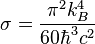$sigma = frac{pi^2 k_B^4}{60 hbar^3 c^2} ;$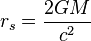$r_s = frac{2GM}{c^2} ;$

The gravitational constant of a black hole at its surface event horizon: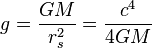$g = frac{G M}{r_s^2} = frac{c^4}{4 G M} ;$

Hawking radiation has a black-body (Planck) spectrum with a temperature T given by:$E = k_B T = frac{hbar g}{2 pi c} = frac{hbar}{2 pi c} left( frac{c^4}{4 G M} right) = frac{hbar c^3}{8 pi G M} ;$$T_H = frac{hbar c^3}{8 pi G M k_B} ;$

Schwarzschild sphere surface area of Schwarzschild radius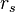$r_s$:$A_s = 4 pi r_s^2 = 4 pi left( frac{2 G M}{c^2} right)^2 = frac{16 pi G^2 M^2}{c^4} ;$

Stefan–Boltzmann power law:$P = A_s j^{star} = A_s epsilon sigma T^{4} ;$

A black hole is a perfect black-body:$epsilon = 1 ;$

Stefan–Boltzmann–Schwarzschild–Hawking black hole radiation power law derivation: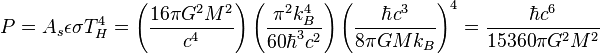$P = A_s epsilon sigma T_H^4 = left( frac{16 pi G^2 M^2}{c^4} right) left( frac{pi^2 k_B^4}{60 hbar^3 c^2} right) left( frac{hbar c^3}{8 pi G M k_B} right)^4 = frac{hbar c^6}{15360 pi G^2 M^2} ;$

Stefan–Boltzmann-Schwarzschild-Hawking power law: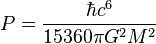$P = frac{hbar c^6}{15360 pi G^2 M^2} ;$

Where$P$ is the energy outflow,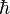$hbar$ is the reduced Planck constant,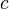$c$ is the speed of light, and$G$ is the gravitational constant. It is worth mentioning that the above formula has not yet been derived in the framework of semiclassical gravity.

The power in the Hawking radiation from a solar mass ($M_{odot}$) black hole turns out to be a minuscule 9 × 10−29 watts. It is indeed an extremely good approximation to call such an object ‘black’.$P = frac{hbar c^6}{15360 pi G^2 M_{odot}^2} = 9.004 times 10^{-29} ; text{W} ;$

Under the assumption of an otherwise empty universe, so that no matter or cosmic microwave background radiation falls into the black hole, it is possible to calculate how long it would take for the black hole to dissipate: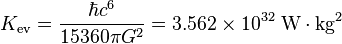$K_{operatorname{ev}} = frac{hbar c^6}{15360 pi G^2} = 3.562 times 10^{32} ; text{W} cdot text{kg}^2 ;$

Given that the power of the Hawking radiation is the rate of evaporation energy loss of the black hole:$P = - frac{dE}{dt} = frac{K_{operatorname{ev}}}{M^2} ;$

Since the total energy E of the black hole is related to its mass M by Einstein’s mass-energy formula:$E = Mc^2 ;$$P = - frac{dE}{dt} = - left( frac{d}{dt} right) M c^2 = -c^2 frac{dM}{dt} ;$

We can then equate this to our above expression for the power: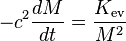$-c^2 frac{dM}{dt} = frac{K_{operatorname{ev}}}{M^2} ;$

This differential equation is separable, and we can write: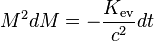$M^2 dM = - frac{K_{operatorname{ev}}}{c^2} dt ;$

The black hole’s mass is now a function M(t) of time t. Integrating over M from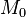$M_0$ (the initial mass of the black hole) to zero (complete evaporation), and over t from zero to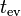$t_{operatorname{ev}} ;$:$int_{M_0}^0 M^2 dM = - frac{K_{operatorname{ev}}}{c^2} int_0^{t_{operatorname{ev}}} dt ;$

The evaporation time of a black hole is proportional to the cube of its mass:$t_{operatorname{ev}} = frac{c^2 M_0^3}{3 K_{operatorname{ev}}} = left( frac{c^2 M_0^3}{3} right) left( frac{15360 pi G^2}{hbar c^6} right) = frac{5120 pi G^2 M_0^3}{hbar c^4} = 8.410 times 10^{-17} left[frac{M_0}{mathrm{kg}}right]^3 mathrm{s} ;$

The time that the black hole takes to dissipate is:$t_{operatorname{ev}} = frac{5120 pi G^2 M_0^{3}}{hbar c^4} ;$

Where$M_0$ is the mass of the black hole.

The lower classical quantum limit for mass for this equation is equivalent to the Planck mass,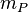$m_P$.

Planck mass quantum black hole Hawking radiation evaporation time: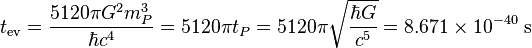$t_{operatorname{ev}} = frac{5120 pi G^2 m_P^3}{hbar c^4} = 5120 pi t_P = 5120 pi sqrt{frac{hbar G}{c^5}} = 8.671 times 10^{-40} ; text{s} ;$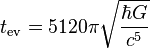$t_{operatorname{ev}} = 5120 pi sqrt{frac{hbar G}{c^5}} ;$

Where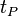$t_P$ is the Planck time.

For a black hole of one solar mass ($M_{odot}$ = 1.98892 × 1030 kg), we get an evaporation time of 2.098 × 1067 years—much longer than the current age of the universe at 13.798 ± 0.037 x 109 years.$t_{operatorname{ev}} = frac{5120 pi G^2 M_{odot}^3}{hbar c^4} = 6.617 times 10^{74} ; text{s} ;$

But for a black hole of 1011 kg, the evaporation time is 2.667 billion years. This is why some astronomers are searching for signs of exploding primordial black holes.

However, since the universe contains the cosmic microwave background radiation, in order for the black hole to dissipate, it must have a temperature greater than that of the present-day black-body radiation of the universe of 2.7 K = 2.3 × 10−4 eV. This implies that$M$ must be less than 0.8% of the mass of theEarth – approximately the mass of the Moon.

Cosmic microwave background radiation universe temperature: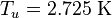$T_u = 2.725 ; text{K} ;$

Hawking total black hole mass:$M_H leq frac{hbar c^3}{8 pi G k_B T_u} leq 4.503 times 10^{22} ; text{kg} ;$$M_H leq frac{hbar c^3}{8 pi G k_B T_u} ;$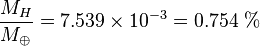$frac{M_H}{M_{oplus}} = 7.539 times 10^{-3} = 0.754 ; % ;$

Where,$M_{oplus}$ is the total Earth mass.

In common units,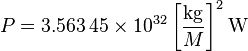$P = 3.563 , 45 times 10^{32} left[frac{mathrm{kg}}{M}right]^2 mathrm{W} ;$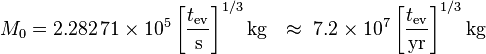$M_0 = 2.282 , 71 times 10^5 left[frac{t_mathrm{ev}}{mathrm{s}}right]^{1/3} mathrm{kg}

approx 7.2 times 10^7 left[frac{t_mathrm{ev}}{mathrm{yr}}right]^{1/3} mathrm{kg} ;$$t_mathrm{ev} = 8.407 , 16 times 10^{-17} left[frac{M_0}{mathrm{kg}}right]^3 mathrm{s}

approx 2.66 times 10^{-24} left[frac{M_0}{mathrm{kg}}right]^3 mathrm{yr} ;$

So, for instance, a 1-second-lived black hole has a mass of 2.28 × 105 kg, equivalent to an energy of 2.05 × 1022 J that could be released by 5 × 106 megatons of TNT. The initial power is 6.84 × 1021 W.

Trinh Do – RCAS